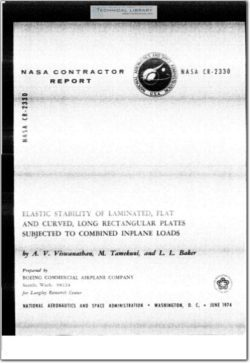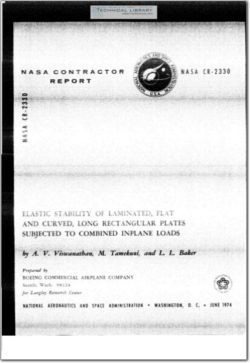17,004 Documents in our Technical Library

AA-CP-20212-001
AA-CP-20212-001
ADPO10769 Occurrence of Corrosion in Airframes
The purpose of this lecture is to provide an overview ...
MIL-STD-1759 Rivets and Rivet Type Fasteners Preferred for Design
The purpose of this book form standard is to provide ...
MIL-STD-810G Environmental Engineering Considerations and Laboratory Tests
This standard contains materiel acquisition program planning and engineering direction ...
MIL-R-7885D General Specification for Rivets, Blind, Structural, Mechanically Locked Spindle and Friction Locked Spindle
This specification covers mechanically locked spindle and  friction locked spindle ...

### NASA-CR-2330• Version
• 2.33 MB File Size
• 1 File Count
• November 27, 2015 Create Date
• November 27, 2015 Last Updated
Scroll for Details

Elastic Stability Of Laminated Flat And Curved Plates.0 SUMMARY

A method is presented to predict theoretical buckling loads of long, rectangular flat and curved
laminated plates with arbitrary orientation of orthotropic axes in each lamina. The plate is
subjected to combined inplane normal and shear loads. Arbitrary boundary conditions may be
stipulated along the longitudinal sides of the plate. In the absence of inplane shear loads and
“extensional-shear” coupling, the analysis is also applicable to finite length plates.

Numerical results are presented for curved laminated composite plates with various boundary
conditions and subjected to various loadings. These results indicate some of the complexities
involved in the numerical solution of the analysis for general laminates. The results also show that
the “reduced bending stiffness” approximation when applied to buckling problems could lead to
considerable error in some cases and therefore must be used with caution.
2.0 INTRODUCTION

Fiber-reinforced composite materials are finding increased applications in aerospace structures.
Capability to predict the buckling behavior of thin laminated plates made from these materials,
when subjected to combined inplane normal and shear loads, is of prime interest to the structural
analyst. A particular characteristic of these laminated plates, in contrast to homogeneous plates, is
the possible coupling between inplane extension and out-of-plane bending, references 1 and 2. Such
coupling can significantly affect the load response of these plates, reference 3.

Considerable literature exists on the buckling of isotropic and orthotropic plates with various
boundary conditions and subjected to various loadings. References 4 through 12 are examples of
analysis for isotropic plates. Reference 13 presents an extensive treatment of orthotropic plates,
where the axes of orthotropy do not coincide with the plate axes, resulting in “mixed-order
derivatives” in the stability equations. A summary of this and other similar work is given in
references 14 and 15.

Buckling of laminated composite plates has been receiving increased attention in the recent
past. References 16 through 21 are examples of the analysis for flat plates. The phenomenon of
possible bending-stretching coupling in these plates is known to have a detrimental effect and adds
to the complexity of the buckling analysis. The use of “reduced bending stiffness” as formulated in
references 1 and 22 has been used in conjunction with classical orthotropic plate buckling analysis,
to allow for coupling effects, references 18, 20, and 21. The inplane boundary conditions do not
enter into this type of analysis. These boundary conditions are known to significantly influence the
buckling of flat plates in the presence of bending-stretching coupling, references 19 and 23.
Therefore, caution has to be exercised in applying the “reduced bending stiffness” concept. As the
number of laminas increases, the exact solution for certain types of lamina layups approaches the
orthotropic plate solution, reference 3.

Few analytical results are available for laminated composite rectangular curved plates. The
analysis of reference 24 may be readily applied to curved plates which are subjected to biaxial
inplane normal loads and wherein no “shear-extensional” coupling is present. For such laminates,
reference 25 considers the effect of the stacking sequence on buckling.

The buckling analysis presented here considers rectangular flat or curved general laminates
subjected to combined inplane normal and shear loads. The analysis is applicable to (i) finite length
plates, when the plate is “specially orthotropic” (i.e., “16” and “26” elements in equations (A—6)
and (A-7) are zero) and the combined inplane loads do not include shear, and (ii) infinitely long
plates, for all other cases. Arbitrary boundary conditions may be specified along the sides (y =
constant). See figure 1. For finite length plates, simply supported boundary conditions are
stipulated along the ends (x = constant).

The method of analysis is such that it may be readily extended to longitudinally stiffened
structures, subjected to combined inplane normal and shear loads, in a manner analogous to that of
reference 24. A stiffness matrix is derived (from a solution of the “stability equations") relating the
buckling displacements and the corresponding forces along the sides (y = constant) of the plate. The
elements of this matrix are transcendental functions of the external loading and the half-wavelength
of buckling, A, in the x-direction. In general, the stiffness matrix is complex and Hermitian in form.
The buckling criterion is formulated in a deterrninantal form, after enforcing the desired boundary
conditions along the sides of the plate. For a chosen half-wavelength, La buckling load is evaluated
by an iteration procedure using the algorithm discussed in reference 26. Therefore a series of
half-wavelengths must be investigated to determine the minimum buckling load.

FileAction
NASA-CR-2330-Elastic-Stability-of-Laminated-Flat-and-Curved-Plates.pdf

### NASA-CR-2330• Version
• 2.33 MB File Size
• 1 File Count
• November 27, 2015 Create Date
• November 27, 2015 Last Updated
Scroll for Details

Elastic Stability Of Laminated Flat And Curved Plates.0 SUMMARY

A method is presented to predict theoretical buckling loads of long, rectangular flat and curved
laminated plates with arbitrary orientation of orthotropic axes in each lamina. The plate is
subjected to combined inplane normal and shear loads. Arbitrary boundary conditions may be
stipulated along the longitudinal sides of the plate. In the absence of inplane shear loads and
“extensional-shear” coupling, the analysis is also applicable to finite length plates.

Numerical results are presented for curved laminated composite plates with various boundary
conditions and subjected to various loadings. These results indicate some of the complexities
involved in the numerical solution of the analysis for general laminates. The results also show that
the “reduced bending stiffness” approximation when applied to buckling problems could lead to
considerable error in some cases and therefore must be used with caution.
2.0 INTRODUCTION

Fiber-reinforced composite materials are finding increased applications in aerospace structures.
Capability to predict the buckling behavior of thin laminated plates made from these materials,
when subjected to combined inplane normal and shear loads, is of prime interest to the structural
analyst. A particular characteristic of these laminated plates, in contrast to homogeneous plates, is
the possible coupling between inplane extension and out-of-plane bending, references 1 and 2. Such
coupling can significantly affect the load response of these plates, reference 3.

Considerable literature exists on the buckling of isotropic and orthotropic plates with various
boundary conditions and subjected to various loadings. References 4 through 12 are examples of
analysis for isotropic plates. Reference 13 presents an extensive treatment of orthotropic plates,
where the axes of orthotropy do not coincide with the plate axes, resulting in “mixed-order
derivatives” in the stability equations. A summary of this and other similar work is given in
references 14 and 15.

Buckling of laminated composite plates has been receiving increased attention in the recent
past. References 16 through 21 are examples of the analysis for flat plates. The phenomenon of
possible bending-stretching coupling in these plates is known to have a detrimental effect and adds
to the complexity of the buckling analysis. The use of “reduced bending stiffness” as formulated in
references 1 and 22 has been used in conjunction with classical orthotropic plate buckling analysis,
to allow for coupling effects, references 18, 20, and 21. The inplane boundary conditions do not
enter into this type of analysis. These boundary conditions are known to significantly influence the
buckling of flat plates in the presence of bending-stretching coupling, references 19 and 23.
Therefore, caution has to be exercised in applying the “reduced bending stiffness” concept. As the
number of laminas increases, the exact solution for certain types of lamina layups approaches the
orthotropic plate solution, reference 3.

Few analytical results are available for laminated composite rectangular curved plates. The
analysis of reference 24 may be readily applied to curved plates which are subjected to biaxial
inplane normal loads and wherein no “shear-extensional” coupling is present. For such laminates,
reference 25 considers the effect of the stacking sequence on buckling.

The buckling analysis presented here considers rectangular flat or curved general laminates
subjected to combined inplane normal and shear loads. The analysis is applicable to (i) finite length
plates, when the plate is “specially orthotropic” (i.e., “16” and “26” elements in equations (A—6)
and (A-7) are zero) and the combined inplane loads do not include shear, and (ii) infinitely long
plates, for all other cases. Arbitrary boundary conditions may be specified along the sides (y =
constant). See figure 1. For finite length plates, simply supported boundary conditions are
stipulated along the ends (x = constant).

The method of analysis is such that it may be readily extended to longitudinally stiffened
structures, subjected to combined inplane normal and shear loads, in a manner analogous to that of
reference 24. A stiffness matrix is derived (from a solution of the “stability equations") relating the
buckling displacements and the corresponding forces along the sides (y = constant) of the plate. The
elements of this matrix are transcendental functions of the external loading and the half-wavelength
of buckling, A, in the x-direction. In general, the stiffness matrix is complex and Hermitian in form.
The buckling criterion is formulated in a deterrninantal form, after enforcing the desired boundary
conditions along the sides of the plate. For a chosen half-wavelength, La buckling load is evaluated
by an iteration procedure using the algorithm discussed in reference 26. Therefore a series of
half-wavelengths must be investigated to determine the minimum buckling load.

FileAction
NASA-CR-2330-Elastic-Stability-of-Laminated-Flat-and-Curved-Plates.pdf
17,004 Documents in our Technical Library